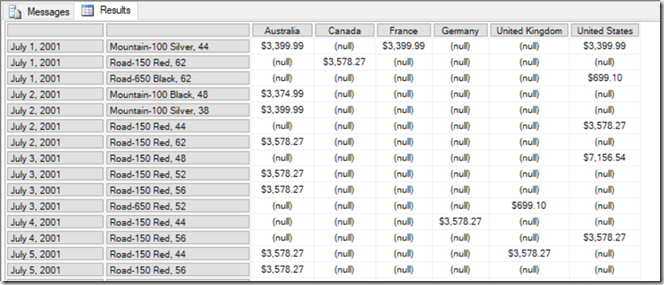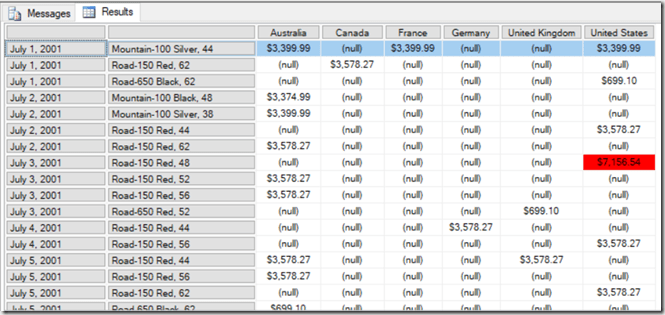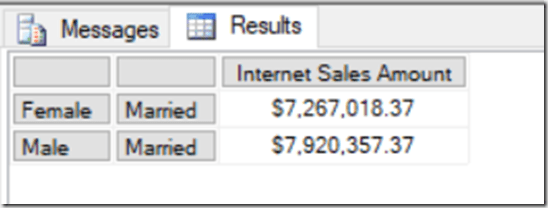# Obscure MDX Month: Optimising MDX That Uses The RGB() Function

In the first blog post in this series a few weeks ago I mentioned that calling Excel and VBA functions from MDX came with a query performance penalty. In this post I’ll give you an illustration of this using the VBA function that I suspect is most frequently called in MDX: the RGB() function.

Take the following MDX query as a baseline:

```WITH
MEMBER MEASURES.TEST AS
[Measures].[Internet Sales Amount]
SELECT {[Customer].[Country].[Country].MEMBERS} ON 0,
NON EMPTY
[Date].[Date].[Date].MEMBERS
*
[Product].[Product].[Product].MEMBERS
ON 1
FROM
WHERE(MEASURES.TEST)
CELL PROPERTIES
VALUE,
FORMATTED_VALUE,
BACK_COLOR
```

It returns Countries on columns and all non empty combinations of Date and Product on rows, and the calculated measure returns the value of the Internet Sales Amount measure:On a SE engine cache it runs in 2.5 seconds on my laptop. With a BACK_COLOR property added to the calculated measure that uses the RGB() function to return the code for red if the measure value is greater than \$5000, query performance is a lot worse: it goes up to 6.5 seconds on a warm SE cache.

```WITH
MEMBER MEASURES.TEST AS
[Measures].[Internet Sales Amount]
,BACK_COLOR=
IIF([Measures].[Internet Sales Amount]>5000,
RGB(255,0,0),
RGB(255,255,255))
SELECT {[Customer].[Country].[Country].MEMBERS} ON 0,
NON EMPTY
[Date].[Date].[Date].MEMBERS
*
[Product].[Product].[Product].MEMBERS
ON 1
FROM
WHERE(MEASURES.TEST)
CELL PROPERTIES VALUE, FORMATTED_VALUE, BACK_COLOR
```That’s a big increase just to do some cell highlighting! However in this case the RGB() function can only return two possible integer values, so if you replace the RGB() function with the integers it returns, like so:

```WITH
MEMBER MEASURES.TEST AS
[Measures].[Internet Sales Amount]
,BACK_COLOR=
IIF([Measures].[Internet Sales Amount]>5000,
255,
16777215)
SELECT {[Customer].[Country].[Country].MEMBERS} ON 0,
NON EMPTY
[Date].[Date].[Date].MEMBERS
*
[Product].[Product].[Product].MEMBERS
ON 1
FROM
WHERE(MEASURES.TEST)
CELL PROPERTIES VALUE, FORMATTED_VALUE, BACK_COLOR
```

…then the query returns in around 3.5 seconds. The last thing to remember is that IIF() statements can perform better if one branch returns null, and in this case we can replace the integer value 16777215 that gives the white background with a null and get the same result:

```WITH
MEMBER MEASURES.TEST AS
[Measures].[Internet Sales Amount]
,BACK_COLOR=
IIF([Measures].[Internet Sales Amount]>5000,
255,
16777215)
SELECT {[Customer].[Country].[Country].MEMBERS} ON 0,
NON EMPTY
[Date].[Date].[Date].MEMBERS
*
[Product].[Product].[Product].MEMBERS
ON 1
FROM
WHERE(MEASURES.TEST)
CELL PROPERTIES VALUE, FORMATTED_VALUE, BACK_COLOR
```

Now the query returns in around 3 seconds, only 0.5 seconds slower than the original with no colour coding.

# Obscure MDX Month: Optimising The Performance Of Total-To-Date Calculations In SSAS Multidimensional

Here’s a SSAS Multidimensional MDX tip that I picked up at the PASS Summit back in 2008 at Mosha’s excellent “MDX Deep Dive” precon (incidentally the slides and supporting material are still available here, although a lot of the material is out of date). It’s regarding total-to-date calculations, ie calculations where you are doing a running total from the very first date you have data for up to the current date. The standard way of writing these calculations is something like this:

```WITH
MEMBER MEASURES.[TTD Sales] AS
SUM(
NULL:[Date].[Calendar].CURRENTMEMBER,
[Measures].[Internet Sales Amount])

SELECT
[Customer].[Country].[Country].MEMBERS
ON 0,
NON EMPTY
[Date].[Calendar].[Date].MEMBERS
*
[Product].[Product].[Product].MEMBERS
ON 1
FROM
WHERE(MEASURES.[TTD Sales])

```

This query runs in around 19.2 seconds on my laptop on a cold cache. However if you rewrite it like this:

```WITH
MEMBER MEASURES.[PTTD SALES] AS
SUM(
NULL:[Date].[Calendar].CURRENTMEMBER.PARENT.PREVMEMBER,
[Measures].[Internet Sales Amount])

MEMBER MEASURES.[TTD Sales] AS
MEASURES.[PTTD SALES]
+
SUM(
[Date].[Calendar].CURRENTMEMBER.FIRSTSIBLING:
[Date].[Calendar].CURRENTMEMBER,
[Measures].[Internet Sales Amount])

SELECT
[Customer].[Country].[Country].MEMBERS
ON 0,
NON EMPTY
[Date].[Calendar].[Date].MEMBERS
*
[Product].[Product].[Product].MEMBERS
ON 1
FROM
WHERE(MEASURES.[TTD Sales])

```

…it runs slightly faster: around 16.1 seconds on a cold cache on my laptop. Of course this is a very big query, and on most normal queries the difference in performance would be much less significant, but it could still be useful. In fact it’s very similar to the kind of tricks people used to optimise the performance of YTD calculations back in the days of SSAS 2000 – the subject of my second-ever blog post from December 2004! The idea here is that instead of summing up a large set of dates, the calculation sums up all the dates in the current month and then all the months from the beginning of time up to and including the previous full month. For YTD and most other something-to-date calculations trick like this are no longer needed, and indeed are counter-productive and will make your calculations slower. However it seems that for total-to-date calculations they can still help performance.

# Obscure MDX Month: Current and CurrentOrdinal

When you are writing an MDX expression, everywhere you use a set you can give that set a name and then reference the name later on. This is known as creating an inline named set, something I have blogged about a few times (see here and here) over the years. When you are iterating over a set using a function like Generate() or Filter(), if you give that set a name you can then use the Current and CurrentOrdinal functions to find out more about the item in the set returned at the current iteration.

Consider the following MDX query on the Adventure Works cube:

```SELECT
{[Measures].[Internet Sales Amount]}
ON 0,
{[Customer].[Gender].[Gender].MEMBERS
*
[Customer].[Marital Status].[Marital Status].MEMBERS}
ON 1
FROM
```

It returns a set of four tuples on rows: every combination of Gender and Marital Status:If you pass the set on rows to the Filter() function and give it a name (for example MySet) you can then use the CurrentOrdinal function to find the 1-based ordinal of the current iteration. This query uses the CurrentOrdinal function to filter the set shown above so only the first and third items in the set are returned:

```SELECT {[Measures].[Internet Sales Amount]} ON 0,
FILTER(
{[Customer].[Gender].[Gender].MEMBERS
*
[Customer].[Marital Status].[Marital Status].MEMBERS}
AS MYSET,
MYSET.CURRENTORDINAL=1 OR
MYSET.CURRENTORDINAL=3)
ON 1
FROM
```With an inline named set you can also use the Current function to return the tuple at the current iteration. Here’s another query that uses the Current function to remove the tuple (Female, Single) from the set:

```SELECT
{[Measures].[Internet Sales Amount]} ON 0,
FILTER(
{[Customer].[Gender].[Gender].MEMBERS
*
[Customer].[Marital Status].[Marital Status].MEMBERS}
AS MYSET,
NOT(
MYSET.CURRENT IS
([Customer].[Gender].&[F],[Customer].[Marital Status].&[S])
)
)
ON 1
FROM
```I won’t pretend that these functions are massively useful, but fans of super-complex MDX will enjoy this vintage post where I used them.

# Obscure MDX Month: Recreating The Star Ratings Measure In MDX Using Excel Functions

I still love MDX, but I’m aware that I blog about it less and less – which is a shame, I know. Therefore I’ve decided that for the next four weeks I’m going to write about some obscure MDX topics that hopefully will make all you SSAS MD diehards out there feel less neglected… even if they don’t have much practical use.

Let’s start off with recreating my ever-popular DAX star-ratings measure in MDX. Well, not exactly pure MDX, but did you know that in MDX you can call some Excel functions (in the same way you can call some VBA functions)? It’s a really, really bad thing to do from a query performance point of view, but it does allow you to do some useful calculations that might otherwise be impossible. Here’s a query on the Adventure Works cube that uses the Excel Rept() and Unichar() functions (functions that do not exist in MDX proper) to recreate my start-ratings measure:

```WITH
MEMBER MEASURES.STARS AS
REPT(
UNICHAR(9733),
CINT([Measures].[Internet Sales Amount]/10000))
+
REPT(
UNICHAR(9734),
10-CINT([Measures].[Internet Sales Amount]/10000))

SELECT {[Measures].[Internet Sales Amount],MEASURES.STARS} ON 0,
ORDER(
[Date].[Date].[Date].MEMBERS,
[Measures].[Internet Sales Amount],
BDESC)
ON 1
FROM